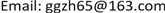CESCreative Education Studies2331-799XScientific Research Publishing10.12677/CES.2019.73062CES-30974CES20190300000_93755639.pdf人文社科 基于校院两级督导制度的教学质量评价指标体系构建研究 Research on the Construction of Teaching Quality Evaluation Index System Based on the Two-Level Supervision System of the University and College 桂桢1*河南工业大学教务处，河南 郑州310520190703351358© Copyright 2014 by authors and Scientific Research Publishing Inc. 2014This work is licensed under the Creative Commons Attribution International License (CC BY). http://creativecommons.org/licenses/by/4.0/1. 引言

2. 校院两级教学督导的组织形式

3. 教学指标体系构建3.1. 建立指标体系

Teaching quality evaluation inde

3.2. 确定权重

1) 建立层次结构模型

2) 构造判断矩阵

Five-dimensional sealin

1因素i与j一样重要
2因素i比j稍微重要
3因素i比j较强重要
4因素i比j强烈重要
5因素i比j绝对重要

E 1 = ( 1 1 / 2 2 1 ) , E 2 = ( 1 1 1 1 ) , E 3 = ( 1 1 / 3 3 1 ) , E 4 = ( 1 1 / 3 3 1 ) , E 5 = ( 1 1 / 2 2 1 ) , E 6 = ( 1 1 1 1 ) , E 7 = ( 1 1 / 2 2 1 ) , E 8 = ( 1 1 / 2 2 1 )

= 2，特征向量为(0.4472, 0.8944)T，对特征向量进行归一化处理，可得(0.333, 0.667)T，接下来结算一致性指标CI，由公式 C I = ( λ max − n ) / ( n − 1 ) 可得，

C I = ( 2 − 2 ) / ( 2 − 1 ) = 0

Mean random consistency inde
n2345
RI0.000.580.901.12

CR = 0 < 0.10，因此指标层的判断矩阵符合一致性要求，因此，第一个专家的B1权重为0.333，B2权重为0.667。

B1 Factor item discriminant matrix, weight and CR valu

C111310.443
C121/311/20.170
C131210.387
λ max = 3.018, CI = 0.009, RI = 0.58, CR = 0.015

B2 Factor item discriminant matrix, weight and CR valu

C21122330.370
C221/211220.208
C231/211210.184
C241/31/21/2110.110
C251/31/21110.128
λ max = 5.055, CI = 0.014, RI = 1.12, CR = 0.013

3.3. 模糊综合评价

1) 建立高等院校教师教学质量评估综合因素评价集合V

V = { B 1 , B 2 } = { 校 级 教 学 督 导 评 价 ， 院 级 教 学 督 导 评 价 }

2) 质量标准化各因素的权重分配，根据上一小节对权重的确定，

3) 校级和院级教学督导教学质量评价等级

Estimation scal

4) 建立评价矩阵Ri

R 1 = ( 0.8 0.1 0.1 0 0 0.9 0.1 0 0 0 0.8 0.2 0 0 0 )

R 2 = ( 0.9 0.1 0 0 0 0.9 0.1 0 0 0 0.8 0.2 0 0 0 0.8 0.2 0 0 0 0.9 0.1 0 0 0 )

5) 求各因素评价矩阵

B 1 = C 1 × R 1 = ( 0.443 0.170 0.387 ) × R 1 = ( 0.8 0.1 0.1 0 0 0.9 0.1 0 0 0 0.8 0.2 0 0 0 )

6) 建立总评价矩阵B

B = ( 0.817 0.139 0.044 0 0 0.871 0.129 0 0 0 )

7) 求风险评价矩阵C

C = A × B = ( 0.354 0.646 ) ( 0.817 0.139 0.044 0 0 0.871 0.129 0 0 0 ) = ( 0.852 0.133 0.016 0 0 )

8) 对工程造价风险评价矩阵C进行归一化处理

C = ( 0.851 0.133 0.016 0 0 )

9) 求系统总得分

f = C 1 × S 1 + C 2 × S 2 + C 3 × S 3 + C 4 × S 4 + C 5 × S 5 = 0.851 × 90 + 0.133 × 80 + 0.016 × 70 + 0 × 60 + 0 × 50 = 88.35

4. 建议

5. 结论

2017年度河南省高等教育教学改革研究与实践一般项目(2017SJGLX300)。